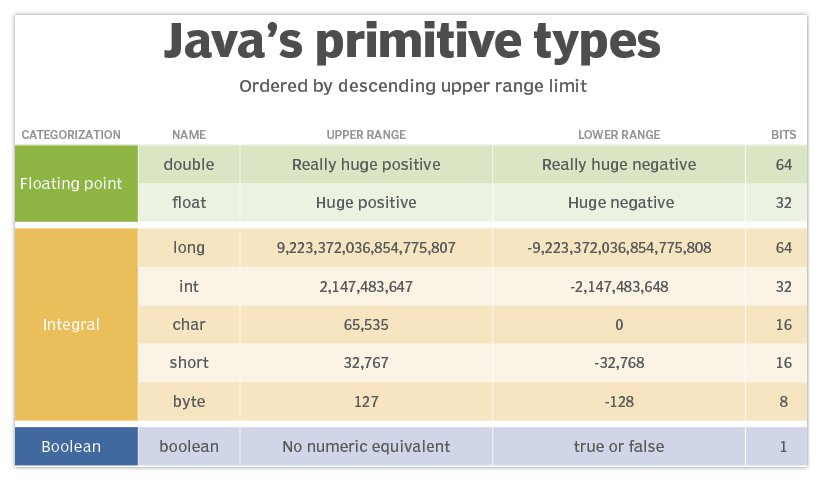# Java double vs float: What's the difference?

## Difference between double and float Java types

The key difference between a float and double in Java is that a double can represent much larger numbers than a float. Both data types represent numbers with decimals, but a float is 32 bits in size while a double is 64 bits. A double is twice the size of a float — thus the term double.

## How do you find the range of a float or double?

In Java, the Float and Double wrapper classes have two properties that return the upper and lower limits of float and double data types, MIN_VALUE and MAX_VALUE:

```System.out.println(Double.MAX_VALUE);
System.out.println(Double.MIN_VALUE);
System.out.println(Float.MAX_VALUE);
System.out.println(Float.MIN_VALUE);```

Some processors and operating systems implement the JVM differently, so different platforms may have some discrepancy between the range of a double or float.

## What is the size difference between float and double Java types?

From the output of the program above, we see the size difference between float and double Java types is:

1. The upper range of a double in Java is 1.7976931348623157E308.
2. The lower range of a double in Java is 4.9E-324.
3. The upper range of a float in Java is 3.4028235E38.
4. The lower range of a float in Java is 1.4E-45.

## How do you declare floats and doubles in Java?

To declare a double in Java, do the following:

• Specify the type as double.
• Provide a variable name.
• Assign a value within the range of a double.
`double trouble = 123.456;`

And here’s how to declare a float in Java:

• Specify the type as float.
• Provide a variable name.
• Assign a value within the range of a float.
• Append the letter f after the last number:
`float some = 654.321f; //note the explicit notation with the letter 'f'`

## Is double or float Java’s default type for decimals?

When Java encounters a decimal in a program, or a calculation generates a floating point number, Java treats the number as a double.

For example, both of the following lines of code fail to compile because Java assumes that any decimal is a double. Since a float can’t handle the full precision of a double, these three lines of code error out:

```float myboat = 1.2;
float yourBoat = 10 * 2.5;
float theBoat = Math.PI * 10;```

## How do you fix double to float conversion errors?

The error, Type mismatch: cannot convert from double to float, can be fixed the following ways:

• Append the letter f to explicitly denote the float.
• Change the data type of myBoat from float to double.
• Use a cast to convert the returned double to a float.
```float myboat = 1.2f;  //explicit notation
double yourBoat = 10 * 2.5;  //change from float to double
float theBoat = (float) (Math.PI * 10);  // explicit cast from double to float```
Difference between float and double Java types
float double
Upper limit 3.4028235E38 1.7976931348623157E308
Lower limit 1.4E-45 4.9E-324
Size 32 bits 64 bits
Explicit notation The letter f (.01f) The letter d (0.1d)
Common uses Microdevices, IoT, mobile Enterprise apps, JPA, JDBC
Java default false true
Alternate BigDecimal BigDecimal
Matching integer type 32-bit int 64-bit long
Wrapper class Float (Upper case ‘F’) Double (Upper case ‘D’)

## When do you use a float vs a double?

In everyday programming, use a double instead of a float.

The double is easier to work with when you are learning Java, and it is most commonly used in enterprise applications.

When memory is limited, such as applications that get deployed to handheld devices, IoT devices or mobile apps, use a float versus a double.

There are also times in data warehousing or AI systems where an application holds tens of thousands of values in an array. If all of the values fall within the range of a float, it makes sense to use the smaller Java data type in this situation, otherwise the array elements needlessly consume memory.

## Are there float and double precision problems in math?

Data precision can be a problem with double and float types because binary numbers don’t always convert cleanly to decimals.

For example, the following program prints out 11.3999 as the sum of 5.6 + 5.8:

```double precision = 0;
precision = precision + 5.6;
precision = precision + 5.8;
System.out.println(precision); // prints 11.399999999999999```

To avoid errors with precision, Java provides a class called BigDecimal that performs floating notation arithmetic with greater precision.Java’s float and double data types are the only two floating point primitive types in the API.

• How to test a predictive model

Strategies for testing predictive models and analytics emphasize data quality, real-time testing and code redundancy, as well as ...

• The dos and don'ts of visual testing

The visual aspect of an application is an important part of UX. Defects can potentially result in lost sales and damaged ...

• 3 QA testing tools to consider

QA testers need to be able to put applications and APIs through their paces. Here are some examples of tools that can help with ...

Close